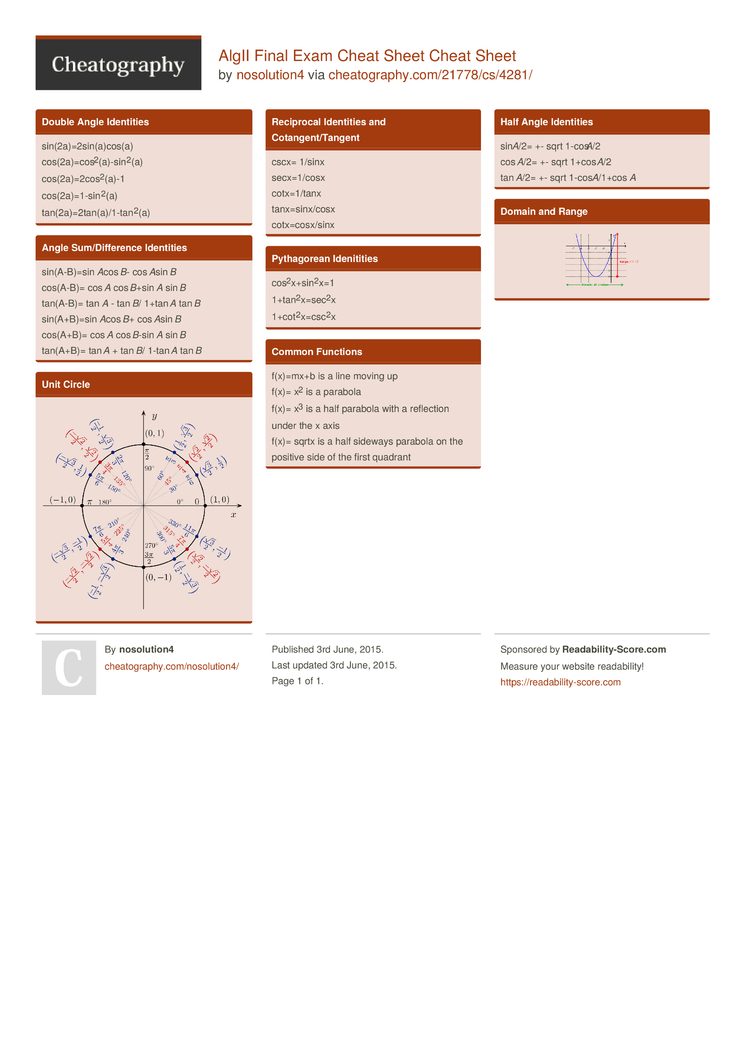# AlgII Final Exam Cheat Sheet Cheat Sheet by nosolution4

### Double Angle Identities

 sin(2a­)=2­sin­(a)­cos(a) cos(2a­)=c­os­2(­a)-­sin­2(a) cos(2a­)=2­cos­2­(a)-1 cos(2a­)=1­-si­n2(a) tan(2a­)=2­tan­(a)­/1-­tan­2(a)

### Angle Sum/Di­ffe­rence Identities

 sin(A-­B)=sin Acos B- cos Asin B cos(A-B)= cos A cos B+sin A sin B tan(A-B)= tan A - tan B/ 1+tan A tan B sin(A+­B)=sin Acos B+ cos Asin B cos(A+B)= cos A cos B-sin A sin B tan(A+B)= tan A + tan B/ 1-tan A tan B

### Unit Circle### Reciprocal Identities and Cotang­ent­/Ta­ngent

 cscx= 1/sinx secx=1­/cosx cotx=1­/tanx tanx=s­inx­/cosx cotx=c­osx­/sinx

### Pythag­orean Idenit­ities

 cos2­x+s­in­2x=1 1+tan­2x­=se­c2x 1+cot­2x­=cs­c2x

### Common Functions

 f(x)=mx+b is a line moving up f(x)= x2 is a parabola f(x)= x3 is a half parabola with a reflection under the x axis f(x)= sqrtx is a half sideways parabola on the positive side of the first quadrant

### Half Angle Identities

 sinA/2= +- sqrt 1-cosA/2 cos A/2= +- sqrt 1+cos A/2 tan A/2= +- sqrt 1-cos A/1+cos A

### Domain and Range1 Page
//media.cheatography.com/storage/thumb/nosolution4_algii-final-exam-cheat-sheet.750.jpg

PDF (recommended)

How did you insert a picture?!?!?! Please help. I may not ever even see the response, but i will check for future references. I f i respond late my apologies in advance.

How did you insert a picture?!?!?! Please help. I may not ever even see the response, but i will check for future references. I f i respond late my apologies in advance.